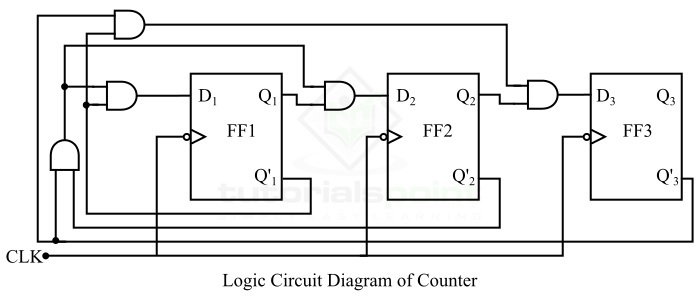# Design of Synchronous Counter

In digital electronics, a set of flip-flops that changes its states in response to pulses applied at the input is called a digital counter. In the digital counter circuit, the flip flops are connected in such a way that their combined state at any time is the binary equivalent of the total number of pulses that have occurred up to that time. Therefore, as its name implies, a counter is used to count pulses in a digital system.

Digital counters are classified into the following two types namely,

• Asynchronous Counter − The type of counter in which the flip flops are connected in such a way that they are not triggered simultaneously is known as asynchronous counter. Asynchronous counter is also known as ripple counter.

• Synchronous Counter − The type of counter in which all the flip flops are clocked simultaneously is known as a synchronous counter.

After getting insights into the basics of counter, now let us discuss the design of synchronous counter.

## Design of Synchronous Counter

A synchronous counter is a type of counter in which all the flip flops are triggered simultaneously by the same clock pulse. The systematic procedure of designing a synchronous counter is explained below.

### Step (1) − Determine the number of flip-flops required

Firstly, analyze the problem description and determine the number of flip-flops required to implement the synchronous counter. If n is the required number of flip flops, then the smallest value of n is such that the number of states N is less than or equal to 2n.

### Step (2) − Draw the state diagram

Secondly, draw the state diagram that shows all the possible states. The state diagram is basically a graphical way of representing the sequence of states through which the counter progresses. In the state diagram, we can also include the case in which the counter goes to a particular state from the invalid states on the next clock pulse.

### Step (3) − Selection of flip-flops and excitation table

In the third step, select a particular type of flip flop to be used to implement the counter and draw its excitation table. The excitation table is one that gives the information about the present states, next states, and required excitations of the flip flop.

### Step (4) − Obtain the minimized expression for excitations

Now, obtain the minimal expressions for the excitations of the flip flops by using any minimization technique such as K-maps.

### Step (5) − Draw the logic diagram

Finally, draw the logic circuit diagram according to the minimal expression obtained in the step 4.

In this way, we can design a synchronous counter

Synchronous counter may suffer from the problem of lock-out, which means they may not be self-starting. A self-starting counter is a type of synchronous counter that will enter to its proper sequence of states regardless of its initial state. We can make a counter self-starting by designing it so that it enters to a particular state whenever it goes to an invalid state.

Now, let us take an example to understand the procedure of designing a synchronous counter.

## Example

Design a synchronous counter using D flip flops that goes through states 0, 1, 2, 4, 0. The unused states must always go to zero on the next clock pulse.

### Solution

This synchronous counter is designed as per the following steps −

Step 1 − Number of flip flops required −

This synchronous counter has four stable states, i.e. 0 (000), 1 (001), 2 (010), 4 (100). But we require three flip flops because it counts 4 (100) as well. Since three flip-flops can count eight states. Thus, the remaining four states, i.e. 3 (011), 5 (101), 6 (110), and 7 (111) are unused states. As per the problem statement, the unused states must go to 0 (000) after the next clock pulse. Therefore, there are no don’t care states.

Step 2 − Draw the state diagram −

The state diagram of the 0, 1, 2, 4, 0, … counter is drawn as shown in the following figure.Step 3 − Chose the type of flip flop and write the excitation table −

The D flip flop is to be used and the excitation table of the counter using D flip flop is written below.

Present State Next State Required Excitation
Q3 Q2 Q1 Q3 Q2 Q1 D3 D2 D1
000001001
001010010
010100100
011000000
100000000
101000000
110000000
111000000

Step 4 − Derive the minimal expression −

From the excitation table, we can see that there is no minimization is possible. Hence, the expressions for the excitations can be directly written from the excitation table itself as follows −

$$D_{1}=Q_{3}^{'}Q_{2}^{'}Q_{1}^{'}$$

$$D_{2}=Q_{3}^{'}Q_{2}^{'}Q_{1}^{'}$$

$$D_{3}=Q_{3}^{'}Q_{2}^{'}Q_{1}^{'}$$

Step 5 − Draw the logic circuit diagram −

The logic circuit diagram of the counter 0, 1, 2, 4, 0,… as per the expressions is shown in the figure below.This is all about how you can design a synchronous counter.

Updated on: 24-Apr-2023

3K+ Views